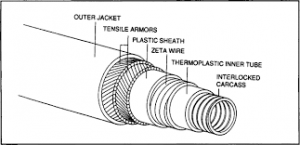## How to Calculate and Solve for Total Load on Flexible Pipe | Irrigation Water RequirementThe image above represents total load on flexible pipe.

To compute for total load on flexible pipe, three essential parameters are needed and these parameters are Load Co-efficient for Ditch Conduits (Cd), Unit Weight of Fill Material (w), Outside Diameter of the Conduit (Bc) and Width of Ditch at Top of Conduit (Bd).

The formula for calculating Total Load on Flexible Pipe:

Wc = CdwBcBd

Where:

Wc = Total Load on Flexible Pipe
Cd = Load Co-efficient for Ditch Conduits
w = Unit Weight of Fill Material
Bc = Outside Diameter of the Conduit
Bd = Width of Ditch at Top of Conduit

Let’s Solve an example;
Find the total load on flexible pipe when the load co-efficient for ditch conduits is 3, the unit weight of fill material is 5, the outside diameter of the conduit is 7 and width of ditch at top of conduit is 5.

This implies that;

Cd = Load Co-efficient for Ditch Conduits = 3
w = Unit Weight of Fill Material = 5
Bc = Outside Diameter of the Conduit = 7
Bd = Width of Ditch at Top of Conduit = 5

Wc = CdwBcBd
Wc = (3)(5)(7)(5)
Wc = 525

Therefore, the total load on flexible pipe is 525.

Calculating for the Load Co-efficient for Ditch Conduits when the Total Load on Flexible Pipe, the Unit Weight of Fill Material, Outside Diameter of the Conduit and the Width of Ditch at Top of Conduit is Given.

Cd = Wc / w x Bc x Bd

Where;

Cd = Load Co-efficient for Ditch Conduits
Wc = Total Load on Flexible Pipe
w = Unit Weight of Fill Material
Bc = Outside Diameter of the Conduit
Bd = Width of Ditch at Top of Conduit

Let’s Solve an example;
Find the load co-efficient for ditch conduits when the total load on flexible pipe is 40, the unit weight of fill material is 4, the outside diameter of the conduit is 6 and the Width of ditch at top of conduit is 2.

This implies that;

Wc = Total Load on Flexible Pipe = 40
w = Unit Weight of Fill Material = 4
Bc = Outside Diameter of the Conduit = 6
Bd = Width of Ditch at Top of Conduit = 2

Cd = Wc / w x Bc x Bd
Cd = 40 / 4 x 6 x 2
Cd = 40 / 48
Cd = 0.83

Therefore, the load co-efficient for ditch conduits is 0.83.